### Areas Class 9th Mathematics AP Board Solution

##### Question 1.In a triangle ABC (see figure), E is the midpoint of median AD, show that(i) ar ∆ABE = ar∆ACE(ii) ar ∆ABE =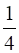ar(∆ABC)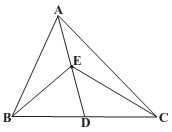Answer:i) Consider ΔABCAD is the median which will divide area(ΔABC) in two equal parts⇒ area(ΔABD) = area(ΔADC) …(i)Consider ΔEBCED is the median which will divide area(ΔEBC) in two equal parts⇒ area(ΔEBD) = area(ΔEDC) …(ii)Subtract equation (ii) from (i) i.e perform equation (i) – equation (ii)⇒ area(ΔABD) - area(ΔEBD) = area(ΔADC) - area(ΔEDC)⇒ area(ΔABE) = area(ΔACE) …(iii)ii) consider ΔABDBE is the median which will divide area(ΔABD) in two equal parts⇒ area(ΔEBD) = area(ΔABE) …(iv)Using equation (iv), (iii) and (ii) we can say thatarea(ΔABE) = area(ΔEBD) = area(ΔEDC) = area(ΔACE) …(v)from figure⇒ area(ΔABC) = area(ΔABE) + area(ΔEBD) + area(ΔEDC) + area(ΔACE)using (v)⇒ area(ΔABC) = area(ΔABE) + area(ΔABE) + area(ΔABE) + area(ΔABE)⇒ area(ΔABC) = 4 × area(ΔABE)⇒ area(ΔABE) =× area(ΔABC)Question 2.Show that the diagonals of a parallelogram divide it into four triangles of equal area.Answer: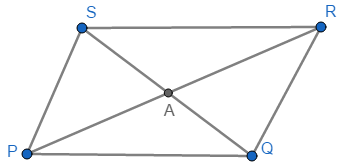Consider parallelogram PQRS whose diagonals intersect at point AProperty of parallelogram is that its diagonal bisect each other⇒ SA = AQ and PA = ARConsider ΔPQSPA is the median which divides the area(ΔPQS) into two equal parts⇒ area(ΔPAS) = area(ΔPAQ) …(i)Consider ΔRQSRA is the median which divides the area(ΔRQS) into two equal parts⇒ area(ΔRAS) = area(ΔRAQ) …(ii)Consider ΔQPRQA is the median which divides the area(ΔQPR) into two equal parts⇒ area(ΔPAQ) = area(ΔRAQ) …(iii)Using equations (i), (ii) and (iii)area(ΔPAS) = area(ΔPAQ) = area(ΔRAQ) = area(ΔRAS)hence, the diagonals of a parallelogram divide it into four triangles of equal areaQuestion 3.In the figure, ∆ABC and ∆ABD are two triangles on the same base AB. If line segment CD is bisected by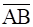at O, show that ar(∆ABC) = ar(∆ABD)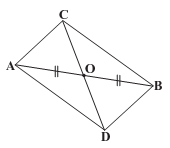Answer:The Figure given in question does not match what the question says here is the correct figure according to the question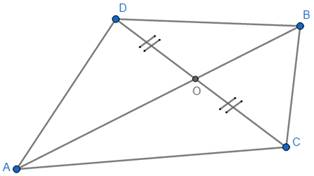Consider ΔACDAO is the median which divides the area(ΔACD) into two equal parts⇒ area(ΔAOD) = area(ΔAOC) …(i)Consider ΔBCDBO is the median which divides the area(ΔBCD) into two equal parts⇒ area(ΔBOD) = area(ΔBOC) …(ii)Add equation (i) and (ii)⇒ area(ΔAOD) + area(ΔBOD) = area(ΔAOC) + area(ΔBOC) …(iii)From figurearea(ΔAOD) + area(ΔBOD) = area(ΔABD) andarea(ΔAOC) + area(ΔBOC) = area(ΔABC)therefore equation (iii) becomesarea(ΔABD) = area(ΔABC)Question 4.In the figure, ∆ABC, D, E, F are the midpoints of sides BC, CA and AB respectively. Show that(i) BDEF is a parallelogram(ii) ar(∆DEF) =ar(∆ABC)(iii) ar(BDEF) =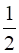ar(∆ABC)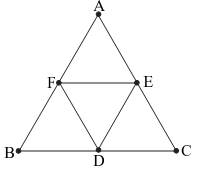Answer:i) consider ΔABCE and F are midpoints of the sides AB and ACThe line joining the midpoints of two sides of a triangle is parallel to the third side and half the third side⇒ EF || BC⇒ EF || BD …(i)And EF =× BCBut D is the midpoint of BC therefore× BC = BD⇒ EF = BD …(ii)E and D are midpoints of the sides AC and BC⇒ ED || AB⇒ ED || FB …(iii)And ED =× ABBut F is the midpoint of AB therefore× AB = FB⇒ ED = FB …(iv)Using (i), (ii), (iii) and (iv) we can say that BDEF is a parallelogramSimilarly we can prove that AFDE and FECD are also parallelogramsii) as BDEF is parallelogram with FD as diagonalthe diagonal divides the area of parallelogram in two equal parts⇒ area(ΔBFD) = area(ΔDEF) …(v)as AFDE is parallelogram with FE as diagonalthe diagonal divides the area of parallelogram in two equal parts⇒ area(ΔAFE) = area(ΔDEF) …(vi)as CEFD is parallelogram with DE as diagonalthe diagonal divides the area of parallelogram in two equal parts⇒ area(ΔEDC) = area(ΔDEF) …(vii)From (v), (vi) and (vii)area(ΔDEF) = area(ΔBFD) = area(ΔAFE) = area(ΔEDC) …(*)from figure⇒ area(ΔABC) = area(ΔDEF) + area(ΔBFD) + area(ΔAFE) + area(ΔEDC)Using (*)⇒ area(ΔABC) = area(ΔDEF) + area(ΔDEF) + area(ΔDEF) + area(ΔDEF)⇒ area(ΔABC) = 4 × area(ΔDEF)⇒ area(ΔDEF) =× area(ΔABC)iii) from figure⇒ area(ΔABC) = area(ΔDEF) + area(ΔBFD) + area(ΔAFE) + area(ΔEDC)Using (*)⇒ area(ΔABC) = area(ΔDEF) + area(ΔBFD) + area(ΔFED) + area(ΔEDC) …(viii)From figurearea(ΔDEF) + area(ΔBFD) = area(BDEF) …(ix)using (*)area(ΔDEF) + area(ΔDEF) = area(BDEF)area(ΔFED) + area(ΔEDC) = area(DCEF) …(x)using (*)area(ΔDEF) + area(ΔDEF) = area(DCEF)therefore area(BDEF) = area(DCEF) …(xi)substituting equation (ix), (x) and (xi) in equation (viii)⇒ area(ΔABC) = area(BDEF) + area(BDEF)⇒ area(ΔABC) = 2 × area(BDEF)⇒ area(BDEF) =× area(ΔABC)Question 5.In the figure D, E are points on the sides AB and AC respectively of ∆ABC such that ar(∆DBC) = ar(∆EBC). Prove that DE || BC.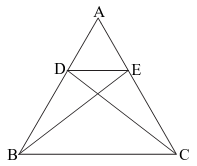Answer: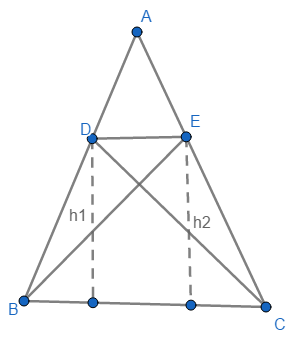Consider h1 and h2 as heights of ∆DBC and ∆EBC from points D and E respectivelyGiven area(∆DBC) = area(∆EBC)The base of both the triangles is common i.e. BCHeight of ∆DBC = h1Height of ∆EBC = h2⇒× BC × h1 =× BC × h2⇒ h1 = h2Which means points D and E are on the same height from segment BC which implies that line passing through both the points i.e. D and E is parallel to the BCTherefore DE || BCQuestion 6.In the figure, XY is a line parallel to BC is drawn through A. If BE || CA and CF || BA are drawn to meet XY at E and F respectively. Show that ar(∆ABE) = ar (∆ACF).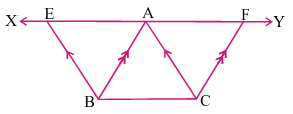Answer:Given XY || BC and BE || CA and CF || BAXY || BC implies EA || BC and AF || BC as points E, A and F lie on XY lineConsider quadrilateral ACBEAC || EB and EA || BC opposite sides are parallelTherefore, quadrilateral ACBE is a parallelogram with AB as the diagonalthe diagonal divides the area of parallelogram in two equal parts⇒ area(ΔABE) = area(ΔABC) …(i)Consider quadrilateral ABCFAB || FC and AF || BC opposite sides are parallelTherefore, quadrilateral ABCF is a parallelogram with AC as the diagonalthe diagonal divides the area of parallelogram in two equal parts⇒ area(ΔACF) = area(ΔABC) …(ii)From (i) and (ii)area(∆ABE) = area(∆ACF)Question 7.In the figure, diagonals AC and BD of a trapezium ABCD with AB || DC intersect each other at O. Prove that ar(∆AOD) = ar(∆BOC).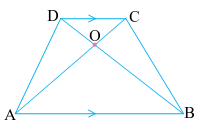Answer:Drop perpendiculars from points D and C on segment AB as shown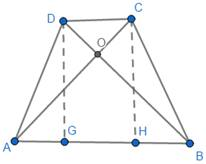Given CD || ABTherefore the perpendicular distance between the parallel lines I equal⇒ DG = CH = hConsider ΔABDBase = ABHeight = GD = hArea(ΔABD) =× AB × h …(i)Consider ΔABCBase = ABHeight = CH = hArea(ΔABC) =× AB × h …(ii)From (i) and (ii)Area(ΔABD) = Area(ΔABC) …(*)Consider ΔAODArea(ΔAOD) = area(ΔABD) - area(ΔABO) …(iii)Consider ΔBOCArea(ΔBOC) = area(ΔABC) - area(ΔABO)But Area(ΔABD) = Area(ΔABC) from (*)⇒ Area(ΔBOC) = area(ΔABD) - area(ΔABO) …(iv)Using (iii) and (iv)Area(ΔAOD) = Area(ΔBOC)Question 8.In the figure, ABCDE is a pentagon. A line through B parallel to AC meets DC produced at F. Show that(i) ar (∆ACB) = ar (∆ACF)(ii) ar (AEDF) = ar (ABCDE)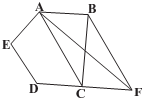Answer:i) Given AC || BFDistance between two parallel lines is constant therefore if we consider AC as common base of ΔABC and ΔFAC then perpendicular distance between lines AC and BF will be same i.e height of triangles ΔABC and ΔFAC will be sameAs ΔABC and ΔFAC are triangles with same base and equal height⇒ area(ΔABC) = area(ΔFAC)ii) since area(ΔABC) = area(ΔFAC)add area(ACDE) to both sides⇒ area(ΔABC) + area(ACDE) = area(ΔFAC) + area(ACDE) …(i)From figurearea(ΔABC) + area(ACDE) = area(ABCDE) …(ii)area(ΔFAC) + area(ACDE) = area(AFDE) …(iii)using (ii) and (iii) in (i)⇒ area(ABCDE) = area(AFDE)Question 9.In the figure, if ar ∆RAS = ar ∆RBS and [ar (∆QRB) = ar(∆PAS) then show that both the quadrilaterals PQSR and RSBA are trapeziums.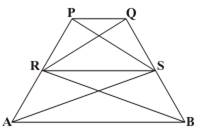Answer:Extend lines R and S to points J and K as shown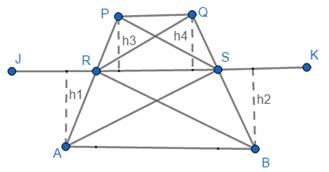Given that area(∆RAS) = area(∆RBS) …(i)Common base is RSLet height of ∆RAS be h1 and ∆RBS be h2 as shownarea(∆RAS) =× RS × h1area(∆RBS) =× RS × h2by given× RS × h1 =× RS × h2⇒ h1 = h2As the distance between two lines is constant everywhere then lines are parallel⇒ RS || AB …(*)Therefore, ABSR is a trapeziumGiven area(∆QRB) = area(∆PAS) …(ii)area(∆QRB) = area(∆RBS) + area(∆QRS) …(iii)area(∆PAS) = area(∆RAS) + area(∆RPS) …(iv)subtract (iii) from (iv)area(∆QRB) - area(∆PAS) = area(∆RBS) + area(∆QRS) - area(∆RAS) - area(∆RPS)using (i) and (ii)⇒ 0 = area(∆QRS) - area(∆RPS)⇒ area(∆QRS) = area(∆RPS)Common base for ∆QRS and ∆RPS is RSLet height of ∆RPS be h3 and ∆RQS be h4 as shownarea(∆RPS) =× RS × h3area(∆RQS) =× RS × h4by given× RS × h3 =× RS × h4⇒ h3 = h4As the distance between two lines is constant everywhere then lines are parallel⇒ RS || PQ⇒ PQSR is a trapeziumQuestion 10.A villager Ramayya has a plot of land in the shape of a quadrilateral. The grampanchayat of the village decided to take over some portion of his plot from one of the corners to construct a school. Ramayya agrees to the above proposal with the condition that he should be given equal amount of land in exchange of his land adjoining his plot so as to form a triangular plot. Explain how this proposal will be implemented. (Draw a rough sketch of plot).Answer:The shape of plot is quadrilateral but actual shape is not mentioned so we can take any quadrilateralHere let us consider shape of plot to be square as shown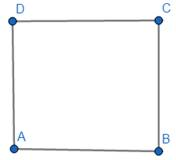Consider O as midpoint of AB and join DO as shown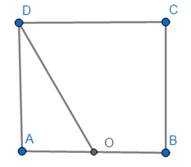Thus AO = OB …(i)Area(ΔAOD) is the area given by ramayya to construct schoolNow extend DO and CB so that they meet at point R as shown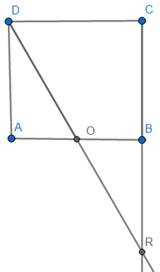Area(ΔBOR) is given to Ramayya so that now his plot is ΔDRCWe have to prove that Area(ΔAOD) = Area(ΔBOR)∠DAO = 90° and ∠OBR = 90° …(ABCD is a square)∠DOA = ∠BOR …(opposite air of angles)By AA criteriaΔDOA ~ ΔROB …(ii)Area(ΔDOA) = 1/2 × DA × OA …(iii)Area(ΔROB) = 1/2 × BR × OBBut from (i) OA = OB⇒ Area(ΔROB) = 1/2 × BR × OA …(iv)Now looking at (iii) and (iv) if we prove DA = BR then it would imply Area(ΔAOD) = Area(ΔBOR)Using (ii)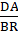=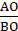But from (i) OA = OB⇒= 1⇒ DA = BR⇒ Area(ΔAOD) = Area(ΔBOR)

PDF FILE TO YOUR EMAIL IMMEDIATELY PURCHASE NOTES & PAPER SOLUTION. @ Rs. 50/- each (GST extra)

HINDI ENTIRE PAPER SOLUTION

MARATHI PAPER SOLUTION

SSC MATHS I PAPER SOLUTION

SSC MATHS II PAPER SOLUTION

SSC SCIENCE I PAPER SOLUTION

SSC SCIENCE II PAPER SOLUTION

SSC ENGLISH PAPER SOLUTION

SSC & HSC ENGLISH WRITING SKILL

HSC ACCOUNTS NOTES

HSC OCM NOTES

HSC ECONOMICS NOTES

HSC SECRETARIAL PRACTICE NOTES

# 2019 Board Paper Solution

HSC ENGLISH SET A 2019 21st February, 2019

HSC ENGLISH SET B 2019 21st February, 2019

HSC ENGLISH SET C 2019 21st February, 2019

HSC ENGLISH SET D 2019 21st February, 2019

SECRETARIAL PRACTICE (S.P) 2019 25th February, 2019

HSC XII PHYSICS 2019 25th February, 2019

CHEMISTRY XII HSC SOLUTION 27th, February, 2019

OCM PAPER SOLUTION 2019 27th, February, 2019

HSC MATHS PAPER SOLUTION COMMERCE, 2nd March, 2019

HSC MATHS PAPER SOLUTION SCIENCE 2nd, March, 2019

SSC ENGLISH STD 10 5TH MARCH, 2019.

HSC XII ACCOUNTS 2019 6th March, 2019

HSC XII BIOLOGY 2019 6TH March, 2019

HSC XII ECONOMICS 9Th March 2019

SSC Maths I March 2019 Solution 10th Standard11th, March, 2019

SSC MATHS II MARCH 2019 SOLUTION 10TH STD.13th March, 2019

SSC SCIENCE I MARCH 2019 SOLUTION 10TH STD. 15th March, 2019.

SSC SCIENCE II MARCH 2019 SOLUTION 10TH STD. 18th March, 2019.

SSC SOCIAL SCIENCE I MARCH 2019 SOLUTION20th March, 2019

SSC SOCIAL SCIENCE II MARCH 2019 SOLUTION, 22nd March, 2019

XII CBSE - BOARD - MARCH - 2019 ENGLISH - QP + SOLUTIONS, 2nd March, 2019

# HSCMaharashtraBoardPapers2020

(Std 12th English Medium)

HSC ECONOMICS MARCH 2020

HSC OCM MARCH 2020

HSC ACCOUNTS MARCH 2020

HSC S.P. MARCH 2020

HSC ENGLISH MARCH 2020

HSC HINDI MARCH 2020

HSC MARATHI MARCH 2020

HSC MATHS MARCH 2020

# SSCMaharashtraBoardPapers2020

(Std 10th English Medium)

English MARCH 2020

HindI MARCH 2020

Hindi (Composite) MARCH 2020

Marathi MARCH 2020

Mathematics (Paper 1) MARCH 2020

Mathematics (Paper 2) MARCH 2020

Sanskrit MARCH 2020

Sanskrit (Composite) MARCH 2020

Science (Paper 1) MARCH 2020

Science (Paper 2)

Geography Model Set 1 2020-2021

MUST REMEMBER THINGS on the day of Exam

Are you prepared? for English Grammar in Board Exam.

Paper Presentation In Board Exam

How to Score Good Marks in SSC Board Exams

Tips To Score More Than 90% Marks In 12th Board Exam

How to write English exams?

How to prepare for board exam when less time is left

How to memorise what you learn for board exam

No. 1 Simple Hack, you can try out, in preparing for Board Exam

How to Study for CBSE Class 10 Board Exams Subject Wise Tips?

JEE Main 2020 Registration Process – Exam Pattern & Important Dates

NEET UG 2020 Registration Process Exam Pattern & Important Dates

How can One Prepare for two Competitive Exams at the same time?

8 Proven Tips to Handle Anxiety before Exams!